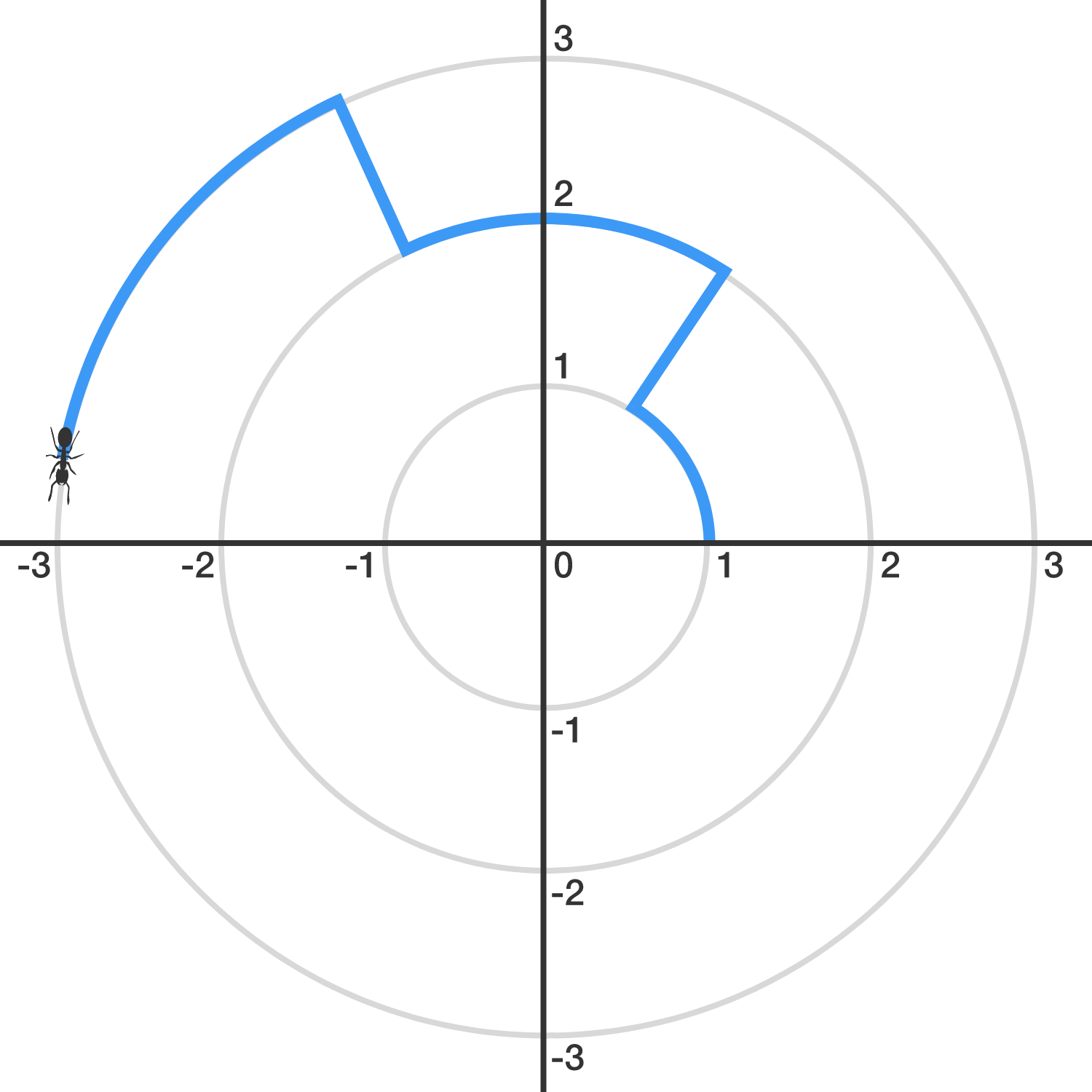Classical Mechanics

# Angular Kinematics: Level 2-3 Challenges

When a ceiling fan is switched off, its angular velocity reduces to half after it makes 36 rotations. How many more rotations will it make before coming to rest?

The angular deceleration of the fan is uniform.An ant finds itself trapped in the $xy$-plane, and its initial position is $(1,0).$

Let $S_k$ denote the circle with radius $k$ centered around the origin. Starting from $(1,0)$, the ant walks 1 unit counter-clockwise on $S_{1}.$ Then, it walks directly (radially outward) to $S_2,$ on which it will walk 2 units counter-clockwise. Then, it will walk directly to $S_3$ and walk 3 units counter-clockwise, and so, with the ant walking $k$ units on $S_k.$ (See the image above.)

When the ant crosses the positive $x$-axis for the first time since it left $(1,0)$, it is on $S_{n}$. What is $n$?

The world record for revolutions per minute for an ice skater is 308 RPM, held by Natalia Kanounnikova. You can see a video of the spin here.

When she starts the spin and her leg is extended, she's going somewhere between 1-2 revolutions per second. Let's average this and say her initial angular velocity is 1.5 revolutions/sec. She has to do work to move her leg in and get herself to speed up. To see this, calculate the ratio of her final rotational kinetic energy to her initial rotational kinetic energy (it's >1 so she had to do some work).

Details and assumptions

• Neglect any friction with the ice.

During the spin cycle of a washing machine, the clothes stick to the outer wall of the barrel as it spins at a rate as high as $1800$ revolutions per minute. The radius of the barrel is $26 \text{ cm.}$ Determine the approximate speed of the clothes (in m/s) which are located on the outer wall of the spinning barrel.A DVD player can exert a torque on a DVD of $10^{-3}~\mbox{N} \cdot \mbox{m}$. What is the DVD’s angular acceleration in $\mbox{rad/s}^2$?

Details and assumptions

• The DVD has a mass of $0.015~\mbox{kg}$ and a radius of $0.06~\mbox{m}$.
• A DVD can be modeled as a thin, uniform cylinder.
×